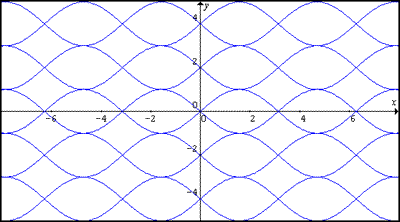#### You may also like### Cubic Spin

Prove that the graph of f(x) = x^3 - 6x^2 +9x +1 has rotational symmetry. Do graphs of all cubics have rotational symmetry?### Parabolic Patterns

The illustration shows the graphs of fifteen functions. Two of them have equations y=x^2 and y=-(x-4)^2. Find the equations of all the other graphs.### More Parabolic Patterns

The illustration shows the graphs of twelve functions. Three of them have equations y=x^2, x=y^2 and x=-y^2+2. Find the equations of all the other graphs.

# Sine Problem

##### Age 16 to 18Challenge LevelIn this image, one of the graphs is that of the sine function. Find the equations of the other graphs to reproduce the pattern.

Many thanks Aditya Sahu from Bristol Grammar School for sending us this puzzle.